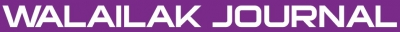### Finding the Exact Solution of Special Nonlinear Partial Differential Equations by Homotopy Analysis Method

#### Abstract

In this paper, the homotopy analysis method was used to solve nonlinear parabolic-hyperbolic partial differential equations. Examples are presented here to show the usability of the method for such equations. The results show that the HAM is very effective and convenient and that the obtained solutions of HAM have high accuracy.

doi:10.14456/WJST.2014.36

#### Keywords

Homotopy analysis method, nonlinear parabolic-hyperbolic partial differential equations

PDF

#### References

SJ Liao. 1992, The proposed homotopy analysis technique for the solution of nonlinear problems. Ph.D. Thesis, Shanghai Jiao Tong University, China.

SJ Liao. Beyond Perturbation: Introduction to the Homotopy Analysis Method. CRC Press, Boca Raton, 2003.

SJ Liao. On the homotopy analysis method for nonlinear problems. Appl. Math. Comput. 2004; 147, 499-513.

M Saeidy, M Matinfar and J Vahidi. Analytical solution of BVPs for fourth-order integro-differential equations by using homotopy analysis method. Int. J. Nonlinear Sci. 2010; 9, 414-21.

M Ayub, A Rasheed and T Hayat. Exact flow of a third grade fluid past a porous plate using homotopy analysis method. Int. J. Eng. Sci. 2003; 41, 2091-103.

JH He. A coupling method of homotopy technique and perturbation technique for nonlinear problems. Int. J. Nonlinear Mech. 2000; 35, 37-43.

M Matinfar and M Saeidy. The homotopy perturbation method for solving higher dimensional initial boundary value problems of variable coefficients. World J. Model. Simulat. 2009; 9, 72-80.

### Refbacks

• There are currently no refbacks.Online ISSN: 2228-835X

http://wjst.wu.ac.th

Last updated: 20 June 2019